# How to Extract the last N words in Excel?

• Last Updated : 24 Jun, 2022

Excel is the tool for storing and managing a large amount of data. Data is entered in a rectangular block which we called a cell. This tool can be used to perform different tasks like creating graphs, and analyzing trends, to get insights from the data. It is primarily used for financial data analysis by accounting professionals. Let’s learn how to extract the last N-words in excel. For example, we have a string “Welcome to GeeksforGeeeks platform” and we need to extract the last 2 words from the string, then the output should be “GeeksforGeeks Platform“.

### Extraction of last N words

For the purpose of demonstration, we will use the following string “Hello Geeks, Welcome to the GeeksforGeeks Platform”. As an example, We will extract the last 5 words of the given string. Follow the below steps,

Formula to extract last N words

Step 1: Count the number of spaces in the string. The formula for counting spaces,

LEN(cell_name)-LEN(SUBSTITUTE(cell_name,” “,””))

Example: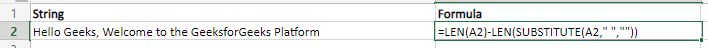Output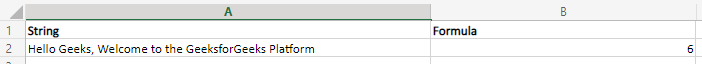Step 2: Replace the fifth space from the last with any special character like @. Formula is,

SUBSTITUTE(cell_name,” “,”@”,(LEN(cell_name)-LEN(SUBSTITUTE(cell_name,” “,””))-5+1)).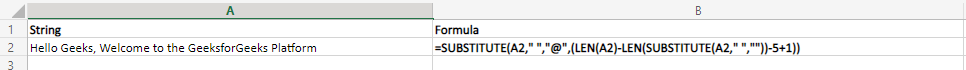Output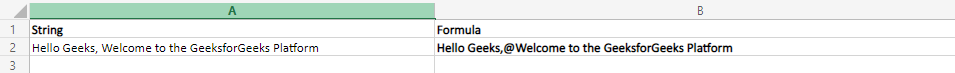Step 3: Get the location of the special character. Formula is,

LEN(cell_name)-FIND(“@”,SUBSTITUTE(cell_name,” “,”@”,(LEN(cell_name)-LEN(SUBSTITUTE(cell_name,” “,””))-5+1)))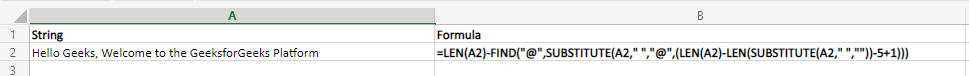Output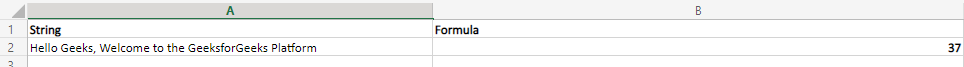Step 4: Extract all the characters after the special characters. Formula is,

RIGHT(cell_name,LEN(cell_name)-FIND(“@”,SUBSTITUTE(cell_name,” “,”@”,(LEN(cell_name)-LEN(SUBSTITUTE(cell_name,” “,””))-5+1))))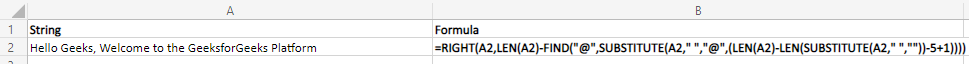Output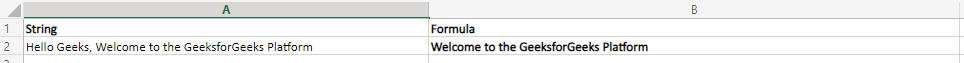So, the final formula to get the last 5 words from the string is,

= RIGHT(cell_name,LEN(cell_name)-FIND(“@”,SUBSTITUTE(cell_name,” “,”@”,(LEN(cell_name)-LEN(SUBSTITUTE(cell_name,” “,””))-5+1))))

Replace 5 with the value of N to get the last N words from the string.

Formula to extract last N words

= RIGHT(cell_name,LEN(cell_name)-FIND(“@”,SUBSTITUTE(cell_name,” “,”@”,(LEN(cell_name)-LEN(SUBSTITUTE(cell_name,” “,””))-N+1))))

My Personal Notes arrow_drop_up
Recommended Articles
Page :